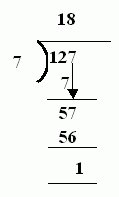Name: ___________________Date:___________________

 Email us to get an instant 20% discount on highly effective K-12 Math & English kwizNET Programs!

### MEAP Preparation - Grade 4 Mathematics1.3 Division With Remainders

 Look at the larger number which is the dividend. The lesser number is called divisor. The dividend is divided by the divisor. If the divisor is not exactly divisible by the divisor, it results in a remainder. Example: 127/718 times 7 gives 126. Answer: 18R1 Directions: Divide the following. Also write at least 10 examples of your own.
 Q 1: Which is the correct answer to 48/9 ?5 R33R56 R 3none of these Q 2: Which is the correct answer to 22/4?3R25R25R1none of these Q 3: Which is the correct answer to 27/5?5R14R35R2none of these Q 4: Which is the correct answer to 75/8?9R38R49R1none of these Q 5: Which is the correct answer to 39/6?none of these6R25R96R3 Q 6: Which is the correct answer to 84/9?none of these7R68R29R3 Q 7: Which is the correct answer to 55/98R16R15R4none of these Q 8: Which is the correct answer to 25/3none of these7R38R17R4 Question 9: This question is available to subscribers only! Question 10: This question is available to subscribers only!